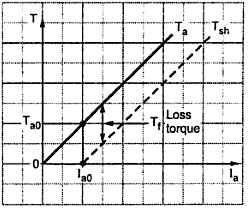### Characteristics of D.C. Shunt Motor

i) Torque - Armature current characteristics
For a d.c. motor                 T α  Φ Ia
For a constant values of Rsh and supply voltage V, Ish is also constant and hence flux is also constant.
..             T α Φ I
The equation represents a straight line, passing through the origin, as shown in the Fig. 1. Torque increases linearly with armature current. It is seen earlier that armature current is decided by the load. So as load increases, armature current increases, increasing the torque developed linearly.Fig. 1  T Vs Ia for shunt motor
Now if shaft torque is plotted against armature current, it is known that shaft torque is less than the armature torque and the difference between the two is loss torque T as shown. On no load Tsh = 0 but armature torque is present which is just enough to overcome stray losses shown as Ta0. The current required is Ia0 on no load to produce Ta0 and hence Tsh graph has an intercept of Ia0 on the current axis.
To generate high starting torque, this type of motor requires a large value of armature current at start. This may damage the motor hence d.c. shunt motors can develop moderate starting torque and hence suitable for such applications where starting torque requirement is moderate.
ii) Speed - Armature current characteristics
From the speed equation we get,
N α (V - I Ra)/Φ
αV - I Ra     as Φ is constant
So as load increases, the armature current increases and hence drop I Ra also increases.
Hence for constant supply voltage, V - I Ra decreases and hence speed reduces. But as  Ra is very small, for change in Ifrom no load to full load, drop Ia Ra is very small and hence drop in speed is also not significant from no load to full load.Fig. 2  N Vs Ia for shunt motor

So the characteristics is slightly droping as shown in the Fig. 2.
But for all practical purposes these type of motors are considered to be a constant speed motors.
iii) Speed - Torque characteristics
These characteristics can be derived from the above two characteristics. This graph is similar to speed-armature current characteristics as torque is proportional to the armature current. This curve shows that the speed almost remains constant through torque changes from no load to full load conditions. This is shown in the Fig. 3.Fig. 3  N Vs T for shunt motor
Related Articles :